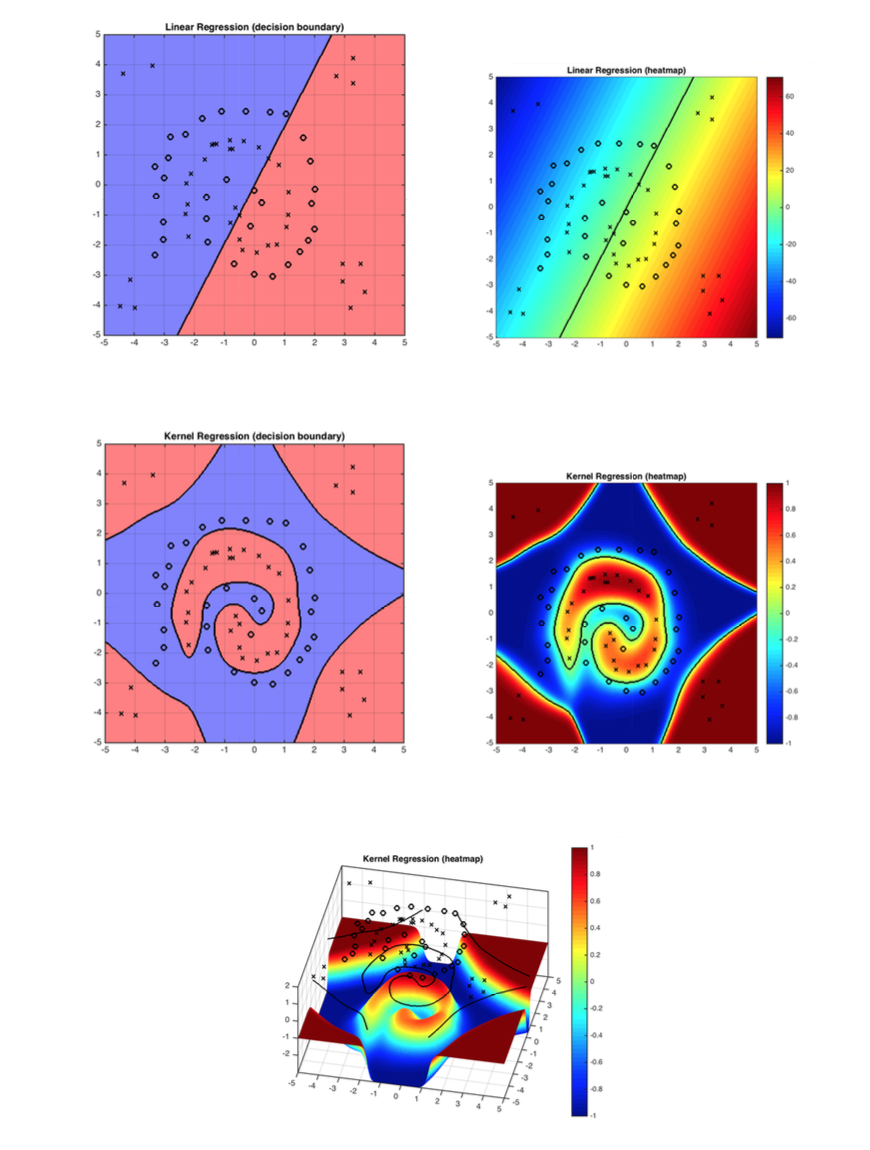## Lecture 13: Kernels

ERM is cool, but so far all classifiers are linear. What if there exists no linear decision boundary? As it turns out, there is an elegant way to incorporate non-linearities into ERM.

### Handcrafted Feature Expansion

We can make classifiers non-linear by applying basis function (feature transformations) on input. For a data vector $\mathbf{x}\in\mathbb{R}^d$, do transformation $\mathbf{x} \rightarrow \phi(\mathbf{x})$ where $\phi(\mathbf{x})\in\mathbb{R}^D$. Usually $D \gg d$ because you add dimensions that capture non-linear interactions among features.

Advantage: It is simple, and your problem stays convex and well behaved. (i.e.you can still use your normal gradient descent descent code)

Disadvantage: $\phi(\mathbf{x})$ might be very high dimensional. (Let's worry about this later)

Consider the following example: $\mathbf{x}=\begin{pmatrix}x_1\\ x_2\\ \vdots \\ x_d \end{pmatrix}$, and define $\phi(\mathbf{x})=\begin{pmatrix}1\\ x_1\\ \vdots \\x_d \\ x_1x_2 \\ \vdots \\ x_{d-1}x_d\\ \vdots \\x_1x_2\cdots x_d \end{pmatrix}$.

Quiz: What is the dimensionality of $\phi(\mathbf{x})$?

So, as is shown above, $\phi(\mathbf{x})$ is very expressive but the dimensionality is extremely high.

### The Kernel Trick

#### Gradient Descent with Squared Loss

Now, note that when we do gradient descent with many loss functions, the gradient is a linear combination of the input samples. Take squared loss for example:

\begin{equation} \ell(\mathbf{w}) = \sum_{i=1}^n (\mathbf{w}^\top \mathbf{x}_i-y_i)^2\label{eq:c15:sql} \end{equation} The gradient descent rule, with step-size $s>0$, updates $\mathbf{w}$ over time, \begin{equation} w_{t+1} \leftarrow w_t - s(\frac{\partial \ell}{\partial \mathbf{w}})\ \textrm{ where: } \frac{\partial \ell}{\partial \mathbf{w}}=\sum_{i=1}^n \underbrace{2(\mathbf{w}^\top \mathbf{x}_i-y_i)}_{\gamma_i\ :\ \textrm{function of $\mathbf{x}_i, y_i$}} \mathbf{x}_i = \sum_{i=1}^n\gamma_i \mathbf{x}_i \end{equation} We will now show that we can express $\mathbf{w}$ as a linear combination of all input vectors, \begin{equation} \mathbf{w}=\sum_{i=1}^n \alpha_i {\mathbf{x}}_i.\label{eq:c15:alphas} \end{equation} Since the loss is convex, the final solution is independent of the initialization, and we can initialize $\mathbf{w}^0$ to be whatever we want. For convenience, let us pick $\mathbf{w}_0=\begin{pmatrix}0 \\ \vdots \\ 0\end{pmatrix}$. For this initial choice of $\mathbf{w}_0$, the linear combination in $\mathbf{w}=\sum_{i=1}^n \alpha_i {\mathbf{x}}_i$ is trivially $\alpha_1=\dots=\alpha_n=0$. We now show that throughout the entire gradient descent optimization such coefficients $\alpha_1,\dots,\alpha_n$ must always exist, as we can re-write the gradient updates entirely in terms of updating the $\alpha_i$ coefficients: \begin{align} \mathbf{w}_1=&\mathbf{w}_0-s\sum_{i=1}^n2(\mathbf{w}_0^\top \mathbf{x}_i-y_i)\mathbf{x}_i=\sum_{i=1}^n \alpha_i^0 {\mathbf{x}}_i-s\sum_{i=1}^n\gamma_i^0\mathbf{x}_i=\sum_{i=1}^n\alpha_i^1\mathbf{x}_i&(\textrm{with $\alpha_i^1=\alpha_i^0-s\gamma_i^0$})\nonumber\\ \mathbf{w}_2=&\mathbf{w}_1-s\sum_{i=1}^n2(\mathbf{w}_1^\top \mathbf{x}_i-y_i)\mathbf{x}_i=\sum_{i=1}^n \alpha_i^1\mathbf{x}_i-s\sum_{i=1}^n\gamma_i^1\mathbf{x}_i=\sum_{i=1}^n\alpha_i^2\mathbf{x}_i&(\textrm{with $\alpha_i^2=\alpha_i^1\mathbf{x}_i-s\gamma_i^1$})\nonumber\\ \mathbf{w}_3=&\mathbf{w}_2-s\sum_{i=1}^n2(\mathbf{w}_2^\top \mathbf{x}_i-y_i)\mathbf{x}_i=\sum_{i=1}^n \alpha_i^2\mathbf{x}_i-s\sum_{i=1}^n\gamma_i^2\mathbf{x}_i=\sum_{i=1}^n\alpha_i^3\mathbf{x}_i&(\textrm{with $\alpha_i^3=\alpha_i^2-s\gamma_i^2$})\nonumber\\ \cdots & \qquad\qquad\qquad\cdots &\cdots\nonumber\\ \mathbf{w}_t=&\mathbf{w}_{t-1}-s\sum_{i=1}^n2(\mathbf{w}_{t-1}^\top \mathbf{x}_i-y_i)\mathbf{x}_i=\sum_{i=1}^n \alpha_i^{t-1}\mathbf{x}_i-s\sum_{i=1}^n\gamma_i^{t-1}\mathbf{x}_i=\sum_{i=1}^n\alpha_i^t\mathbf{x}_i&(\textrm{with $\alpha_i^t=\alpha_i^{t-1}-s\gamma_i^{t-1}$})\nonumber \end{align} The update-rule for $\alpha_i^t$ is thus \begin{equation} \alpha_i^t=\alpha_i^{t-1}-s\gamma_i^{t-1}, \textrm{ and we have } \alpha_i^t=-s\sum_{r=0}^{t-1}\gamma_i^{r}. \end{equation} In other words, we can perform the entire gradient descent update rule without ever expressing $\mathbf{w}$ explicitly. We just keep track of the $n$ coefficients $\alpha_1,\dots,\alpha_n$. Now that $\mathbf{w}$ can be written as a linear combination of the training set, we can also express the inner-product of $\mathbf{w}$ with any input ${\mathbf{x}}_i$ purely in terms of inner-products between training inputs: \begin{equation} \mathbf{w}^\top {\mathbf{x}}_j=\sum_{i=1}^n \alpha_i {\mathbf{x}}_i^\top{\mathbf{x}}_j.\nonumber \end{equation} Consequently, we can also re-write the squared-loss from $\ell(\mathbf{w}) = \sum_{i=1}^n (\mathbf{w}^\top \mathbf{x}_i-y_i)^2$ entirely in terms of inner-product between training inputs: \begin{equation} \ell(\mathbf{\alpha}) = \sum_{i=1}^n \left(\sum_{j=1}^n\alpha_j\mathbf{x}_j^\top \mathbf{x}_i-y_i\right)^2\label{eq:c15:sql:ip} \end{equation} During test-time we also only need these coefficients to make a prediction on a test-input $x_t$, and can write the entire classifier in terms of inner-products between the test point and training points: \begin{equation} h({\mathbf{x}}_t)=\mathbf{w}^\top {\mathbf{x}}_t=\sum_{j=1}^n\alpha_j{\mathbf{x}}_j^\top {\mathbf{x}}_t. \end{equation} Do you notice a theme? The only information we ever need in order to learn a hyper-plane classifier with the squared-loss is inner-products between all pairs of data vectors.

### Inner-Product Computation

Let's go back to the previous example, $\phi(\mathbf{x})=\begin{pmatrix}1\\ x_1\\ \vdots \\x_d \\ x_1x_2 \\ \vdots \\ x_{d-1}x_d\\ \vdots \\x_1x_2\cdots x_d \end{pmatrix}$. The inner product $\phi(\mathbf{x})^\top \phi(\mathbf{z})$ can be formulated as: \begin{equation} \phi(\mathbf{x})^\top \phi(\mathbf{z})=1\cdot 1+x_1z_1+x_2z_2+\cdots +x_1x_2z_1z_2+ \cdots +x_1\cdots x_dz_1\cdots z_d=\prod_{k=1}^d(1+x_kz_k)\text{.}\label{eq:c15:poly} \end{equation} The sum of $2^d$ terms becomes the product of $d$ terms. We can compute the inner-product from above formula in time $O(d)$ instead of $O(2^d)$! In fact, we can pre-compute them and store them in a kernel matrix \begin{equation} \underbrace{\mathsf{K}(\mathbf{x}_i,\mathbf{x}_j)}_{\text{this is called the} \textbf{ Kernel Matrix}}=\phi(\mathbf{x}_i)^\top \phi(\mathbf{x}_j). \end{equation} If we store the matrix $\mathsf{K}$, we only need to do simple inner-product look-ups and low-dimensional computations throughout the gradient descent algorithm.

### General Kernels

Following all some common kernel functions:

Linear: $\mathsf{K}(\mathbf{x},\mathbf{z})=\mathbf{x}^\top \mathbf{z}$.

(The linear kernel is equivalent to just using a good old linear classifier - but it can be faster to use a kernel matrix if the dimensionality $d$ of the data is high.)

Polynomial: $\mathsf{K}(\mathbf{x},\mathbf{z})=(1+\mathbf{x}^\top \mathbf{z})^d$.

Radial Basis Function (RBF) (aka Gaussian Kernel): $\mathsf{K}(\mathbf{x},\mathbf{z})= e^\frac{-\|\mathbf{x}-\mathbf{z}\|^2}{\sigma^2}$.

The RBF kernel is the most popular Kernel! It is a Universal approximator!! Its corresponding feature vector is infinite dimensional.

In the following we provide some other kernels.

Exponential Kernel: $\mathsf{K}(\mathbf{x},\mathbf{z})= e^\frac{-\| \mathbf{x}-\mathbf{z}\|}{2\sigma^2}$

Laplacian Kernel: $\mathsf{K}(\mathbf{x},\mathbf{z})= e^\frac{-| \mathbf{x}-\mathbf{z}|}{\sigma}$

Sigmoid Kernel: $\mathsf{K}(\mathbf{x},\mathbf{z})=\tanh(\mathbf{a}\mathbf{x}^\top + c)$

Think about it: Can any function $\mathsf{K}(\cdot,\cdot)$ be used as a kernel?

No, the matrix $\mathsf{K}(\mathbf{x}_i,\mathbf{x}_j)$ has to correspond to real inner-products after some transformation ${\mathbf{x}}\rightarrow \phi({\mathbf{x}})$. This is the case if and only if $\mathsf{K}$ is positive semi-definite.

Definition: A matrix $A\in \mathbb{R}^{n\times n}$ is positive semi-definite iff $\forall \mathbf{q}\in\mathbb{R}^n$, $\mathbf{q}^\top A\mathbf{q}\geq 0$.

Why is that?

Remember $\mathsf{K}(\mathbf{x},\mathbf{z})=\phi(\mathbf{x})^\top \phi(\mathbf{z})$. A matrix of form $A=\begin{pmatrix} \mathbf{x}_1^\top \mathbf{x}_1, ..., \mathbf{x}_1^\top \mathbf{x}_n \\ \vdots ~ \vdots \\ \mathbf{x}_n^\top \mathbf{x}_1, ..., \mathbf{x}_n^\top \mathbf{x}_n \end{pmatrix}=\begin{pmatrix} \mathbf{x}_1\\ \vdots \\ \mathbf{x}_n \end{pmatrix} \begin{pmatrix} \mathbf{x}_1, \cdots \mathbf{x}_n \end{pmatrix}$ must be positive semi-definite because: $\mathbf{q}^\top A\mathbf{q}=(\underbrace{\begin{pmatrix} \mathbf{x}_1, \cdots \mathbf{x}_n \end{pmatrix}\mathbf{q}}_{\text{a vector with the same dimension of } \mathbf{x}_i})^\top (\begin{pmatrix} \mathbf{x}_1, \cdots \mathbf{x}_n \end{pmatrix}\mathbf{q})\geq 0$ for $\forall \mathbf{q}\in\mathbb{R}^n$.

You can even define kernels over sets, strings, graphs and molecules.Figure 1: The demo shows how kernel function solves the problem linear classifiers can not solve. RBF works well with the decision boundary in this case.